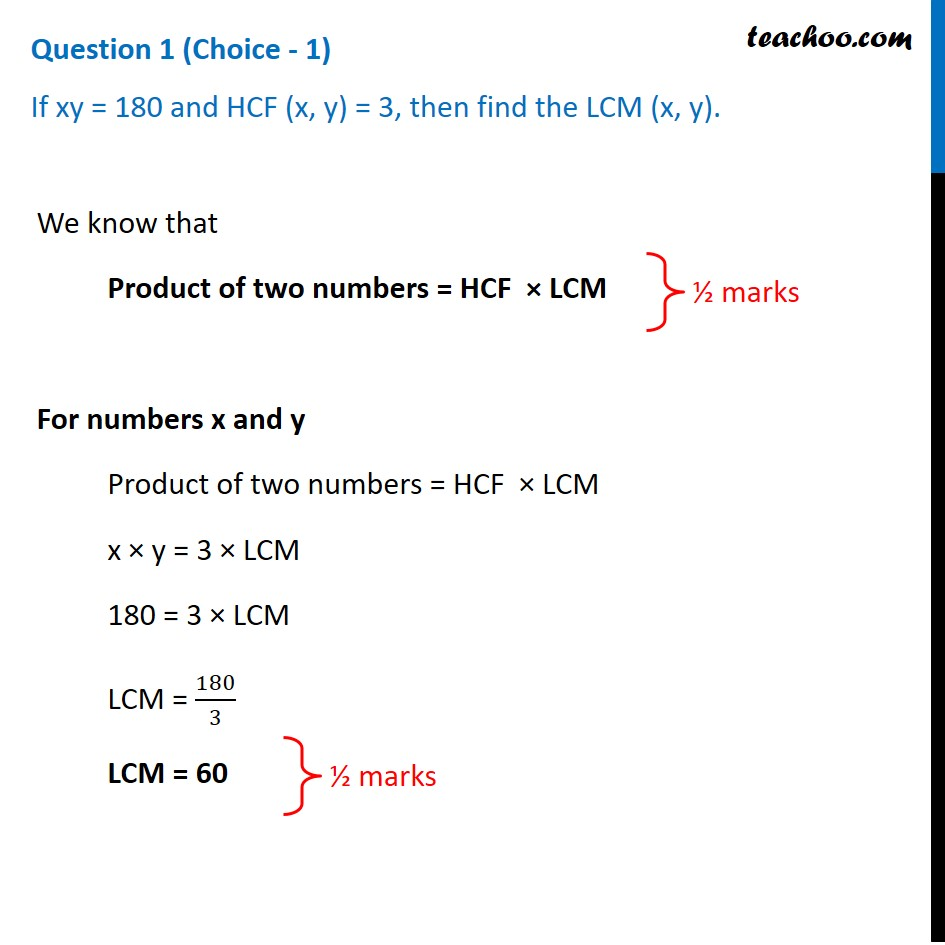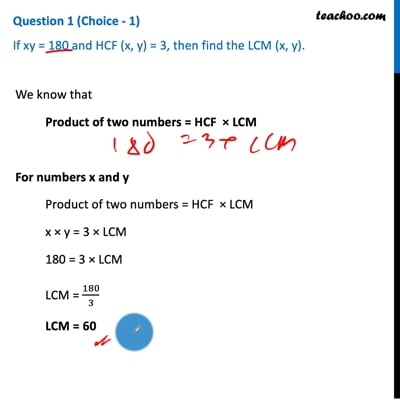CBSE Class 10 Sample Paper for 2021 Boards - Maths Standard

Class 10
Solutions of Sample Papers for Class 10 Boards

## If xy = 180 and HCF (x, y) = 3, then find the LCM (x, y).This video is only available for Teachoo black users

Solve all your doubts with Teachoo Black (new monthly pack available now!)

### Transcript

Question 1 (Choice - 1) If xy = 180 and HCF (x, y) = 3, then find the LCM (x, y). We know that Product of two numbers = HCF × LCM For numbers x and y Product of two numbers = HCF × LCM x × y = 3 × LCM 180 = 3 × LCM LCM = 180/3 LCM = 60